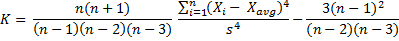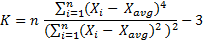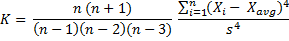# Kurtosis in Excel (KURT)

This page explains the Excel function KURT, which calculates sample excess kurtosis, and how to convert its result to other types of kurtosis (population, sample, non-excess kurtosis).

## KURT Excel Function

In Excel, kurtosis can be comfortably calculated using the KURT Excel function. The only argument needed for KURT function is the range of cells containing the data.

For example the formula:

``=KURT(C5:C104)``

... calculates kurtosis for the set of values contained in cells C5 through C104.

## Which Kind of Kurtosis Excel Actually Calculates

The KURT Excel function calculates sample excess kurtosis – it is this formula:If you want to use Excel for calculating one of the other kinds of kurtosis – sample kurtosis, population kurtosis, or population excess kurtosis, there is no built-in Excel function you can simply use. You can either calculate them by adjusting from the KURT Excel function, or calculate them directly from your input data. See the rest of this page for details.

For differences in the four kinds of kurtosis and detailed explanation of the formulas, see kurtosis formula.

## Calculating Population Excess Kurtosis in Excel

You can calculate population excess kurtosis directly using this formula:One disadvantage of this calculation is that you need to calculate the individual deviations from the mean, squared and raised to the power of 4, in separate cells. If you are working with large data sets, it will make the Excel file very big and possibly slow to calculate.

Therefore it is often better to use the KURT Excel function and adjust the result from sample to population. The adjustment is as follows:

``=((KURT(Data!\$B\$16:\$B\$10015)*(\$G\$5-2)*(\$G\$5-3)/(\$G\$5-1))-6)/(\$G\$5+1)``

This is the function I use in the Descriptive Statistics Calculator. The first part - `KURT(Data!\$B\$16:\$B\$10015)` – is the built-in Excel KURT function for sample excess kurtosis of cells B16 through B10015, and the rest is the adjustment from sample to population excess kurtosis, where cell G5 calculates population size:

``=COUNT(Data!\$B\$16:\$B\$10015)``

The whole formula is:

Population Excess Kurtosis = ( ( Sample Excess Kurtosis · (n – 2) (n – 3) / (n – 1) ) – 6 ) / (n + 1)

... where n = population size (number of values)

As you can see, you don't need to calculate the deviations from the mean in separate cells.

## Calculating Population Kurtosis in Excel

If you want to calculate population (non-excess) kurtosis in Excel, you simply take population excess kurtosis that we've just calculated above and add 3, because:

Population Excess Kurtosis = Population Kurtosis – 3

## Calculating Sample Kurtosis in Excel

Contrary to popular belief, sample excess kurtosis does not equal sample kurtosis less 3 (as it is with population kurtosis), but you need to adjust the minus 3 for the data set being a sample.

Sample excess kurtosis is:Sample kurtosis is:Therefore you can calculate sample kurtosis from sample excess kurtosis (which is the direct result of the built-in KURT Excel function) as follows:

Sample Kurtosis = Sample Excess Kurtosis + ( 3 (n – 1)2 / ( (n – 2) (n – 3) ) )

If you don't mind a large Excel file you can also calculate sample kurtosis directly using the sample kurtosis formula above.

You can see how kurtosis Excel calculation works in practice in the Descriptive Statistics Calculator.

By remaining on this website or using its content, you confirm that you have read and agree with the Terms of Use Agreement.

We are not liable for any damages resulting from using this website. Any information may be inaccurate or incomplete. See full Limitation of Liability.

Content may include affiliate links, which means we may earn commission if you buy on the linked website. See full Affiliate and Referral Disclosure.

We use cookies and similar technology to improve user experience and analyze traffic. See full Cookie Policy.